Introduction to Tableau and Data Visualization
)}

# Data Over Time --- Tableau Implementation

We will now use line charts to analyze the monthly profits of each category. The plot we will build is the following: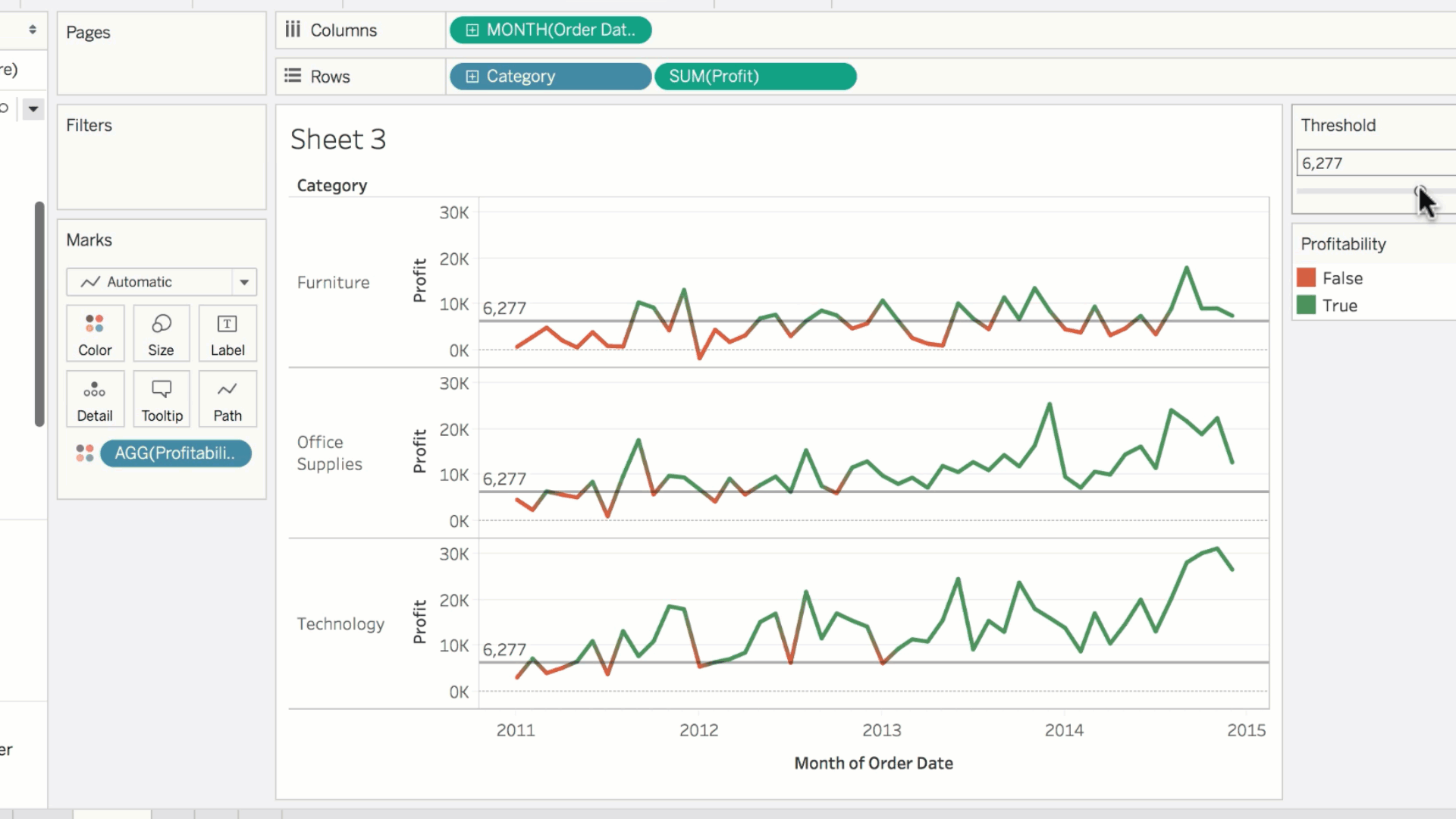The categories are organized in rows; so we drag categories to rows. Each category has its own profit measure spanning the columnar axis; so we drag profit to columns.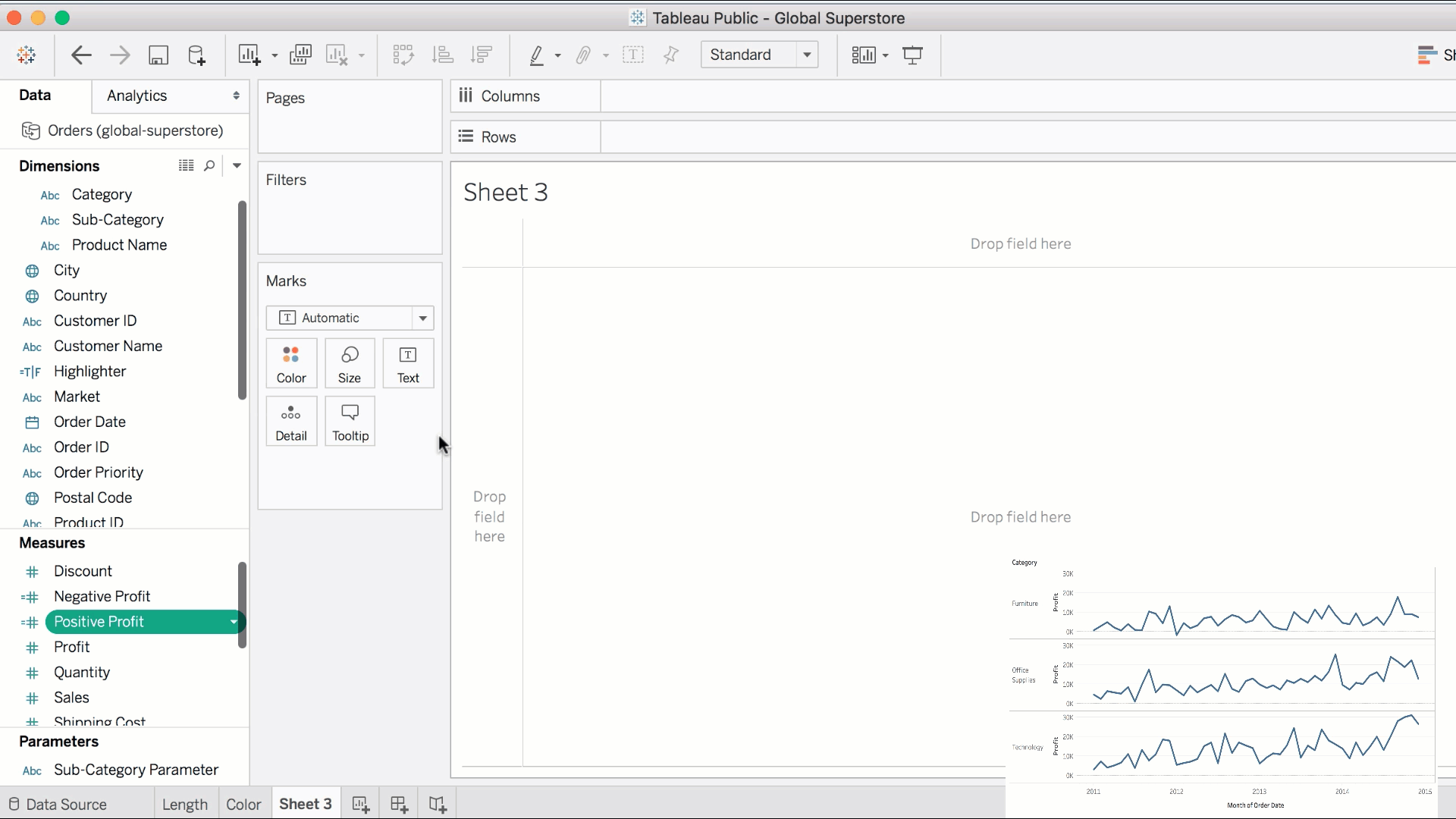This aggregates the sum of profits for each category into a bar. As we wish to analyze the profits over time, we will drag the order date to columns.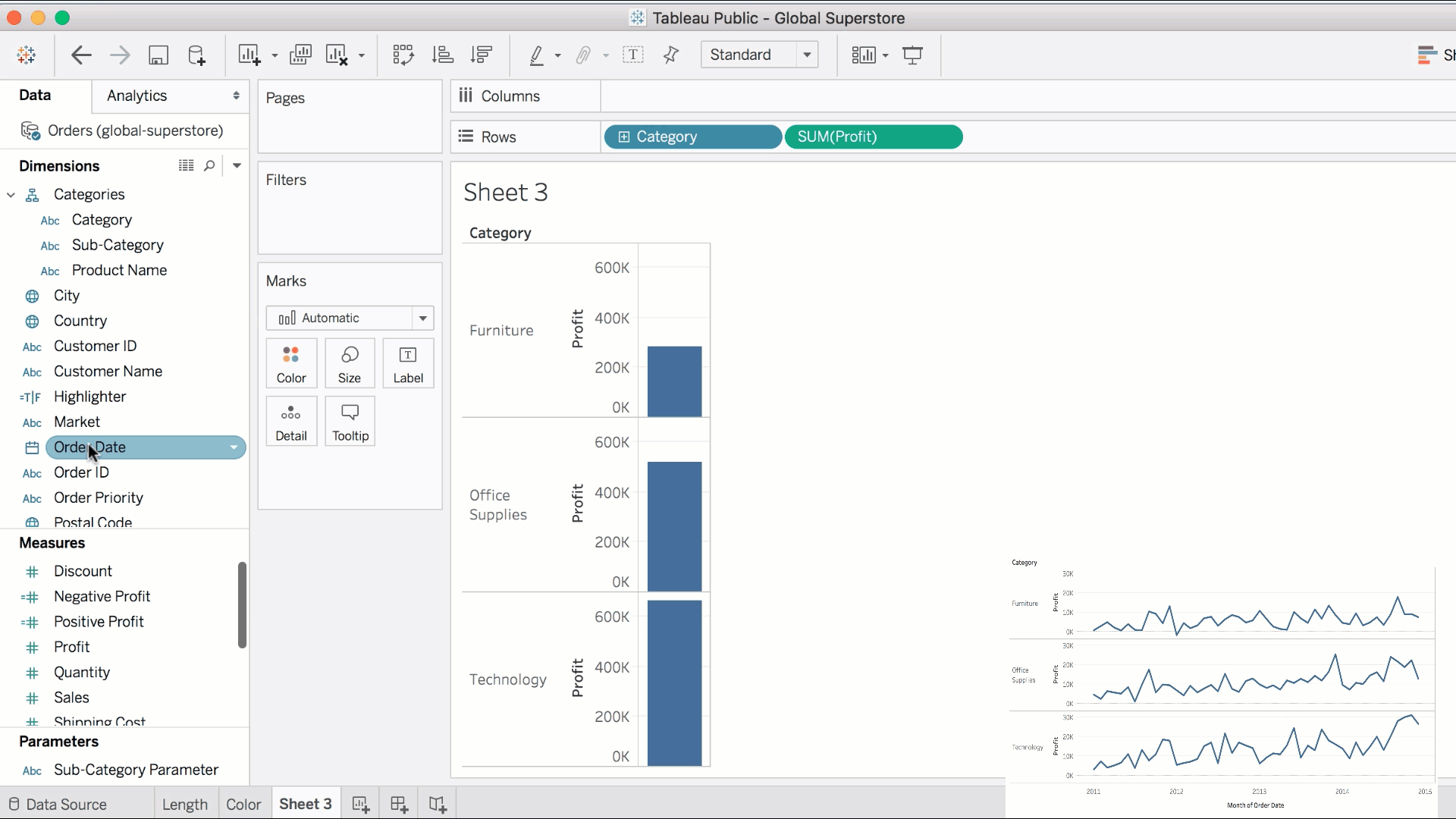The granularity is increased to calculate the sum of profit for each category, at a specific year. Tableau's default for displaying time data is a line graph. You'll also notice that Tableau automatically creates a hierarchy for date fields. To see the profits by month, simply drill down into the hierarchy.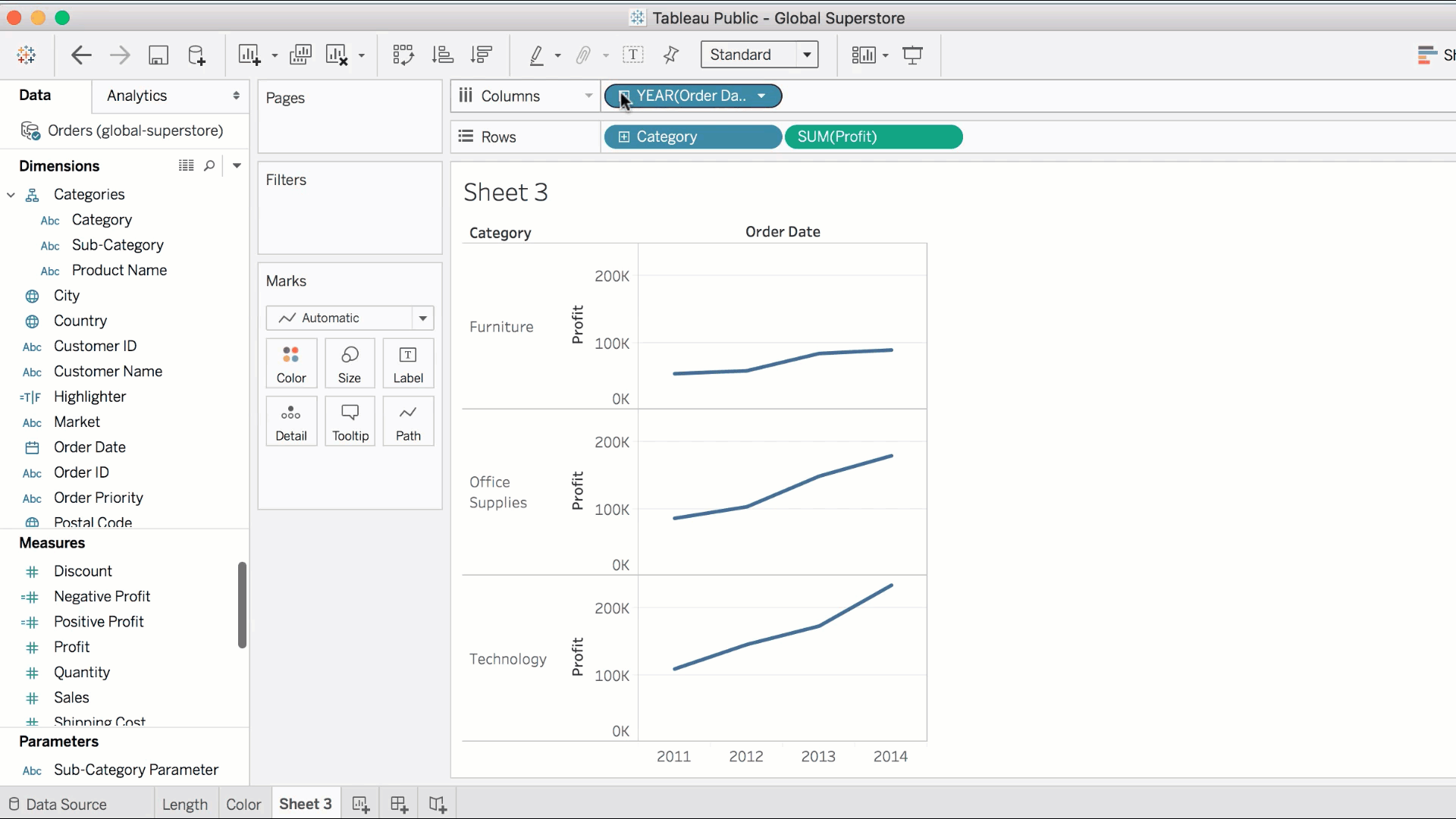The current visual is a little hard to read. Each year is divided into 4 quarters, and each quarter is divided into 3 months. We can omit levels of the hierarchy by simply dragging it off the shelf, and leaving only the months.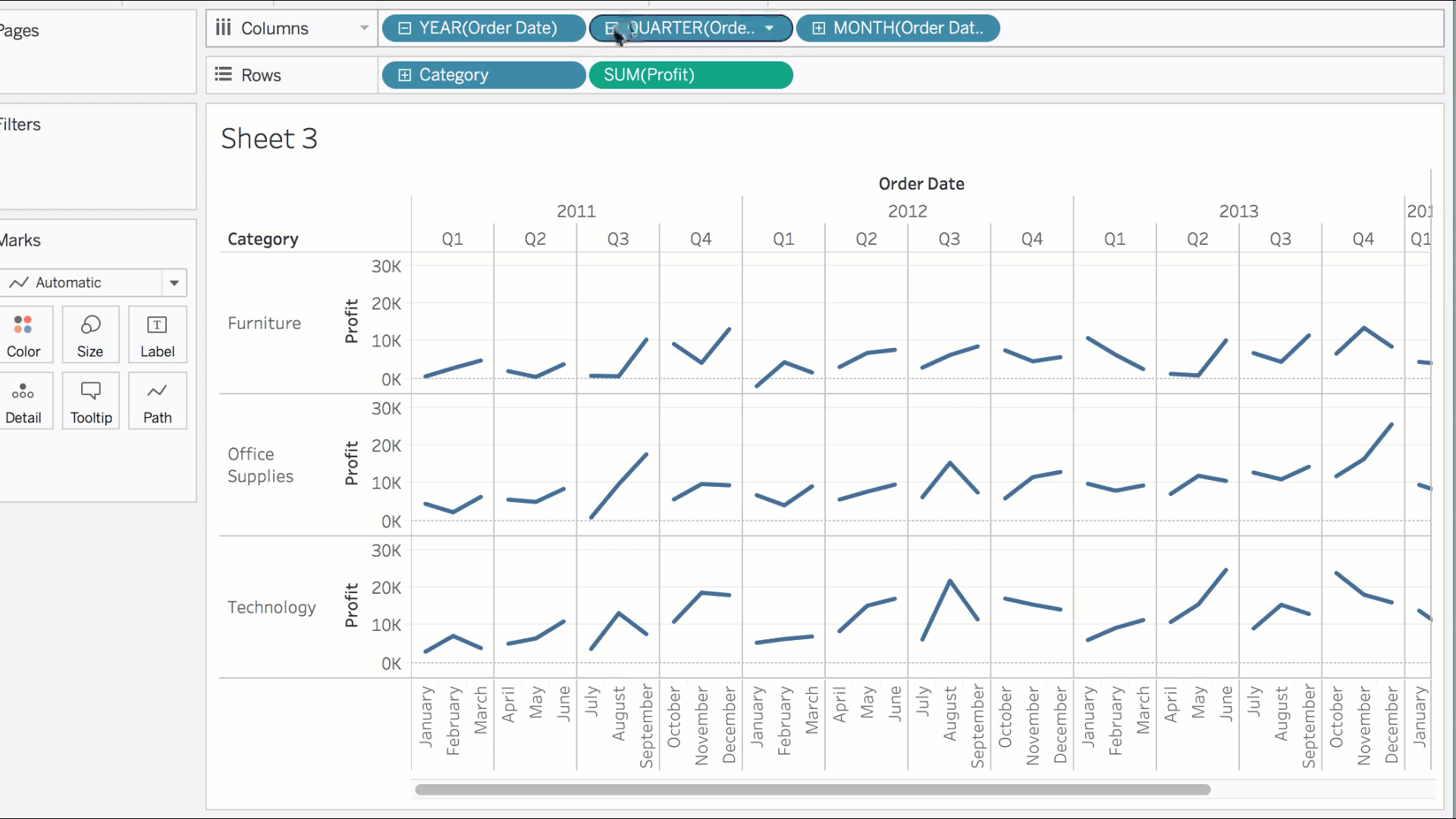Looking at the resulting plot, you might be asking yourself: why is there only one value per month?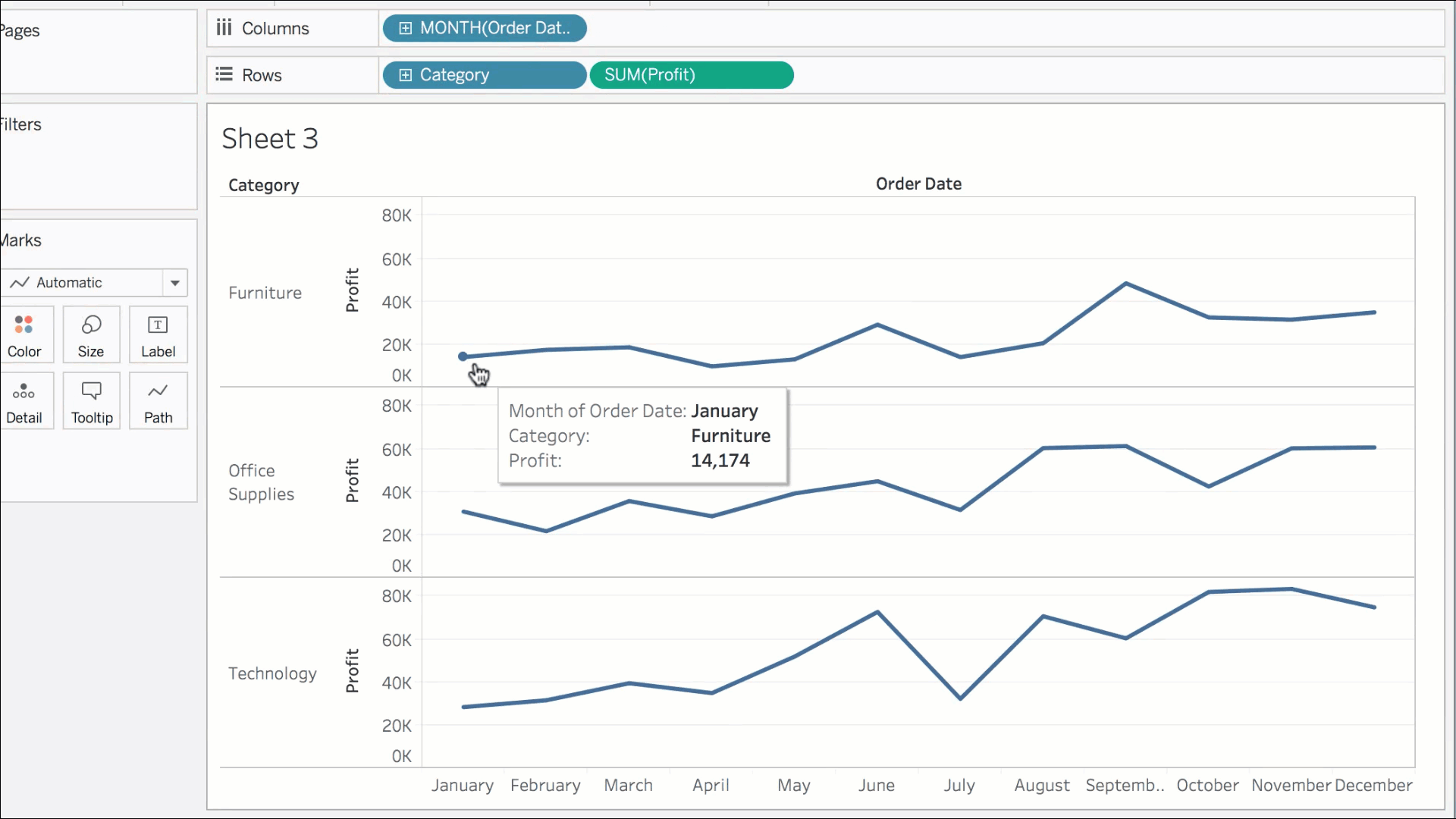Date dimensions can be discrete, or continuous. Right now order date is a discrete dimension (since it's blue). We need it to be continuous. Why?

Think about their definitions:

Discrete means "individually separate and distinct."

Continuous means "forming an unbroken whole, without interruption";

For example, a discrete date will treat the month "January" as its own category, irrespective of the year; thus aggregating the January profits across all 4 years into a single month.

A continuous date will differentiate between months in 2011, 2012, and so on... allowing the line graph to span the entire 4 years in a continuous manner.

Click the drop-down arrow, and choose the **second **instance of month. Ultimately, this will display monthly profits in a continuous manner.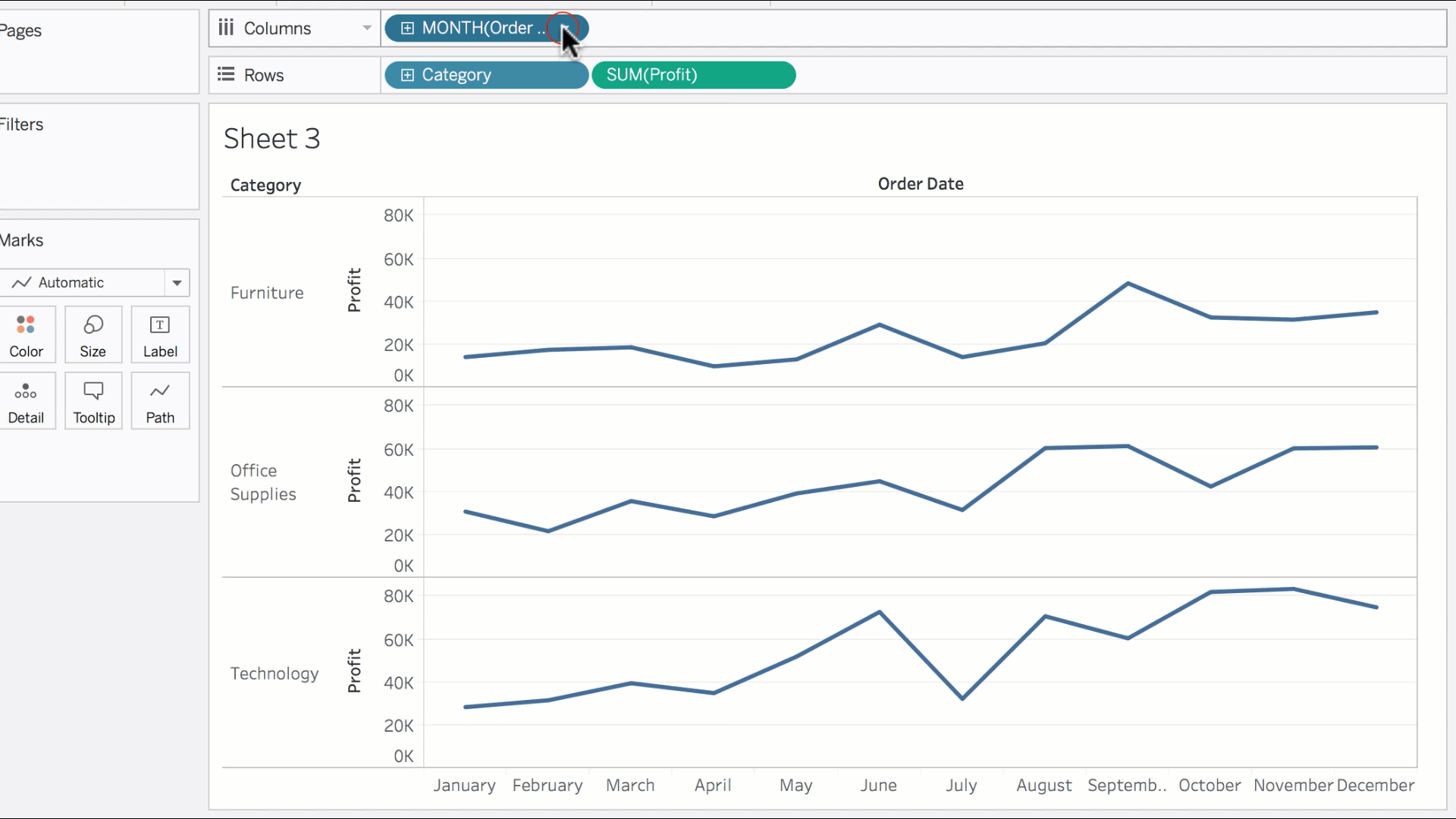Notice that the field is now green! This is the rare example of a **continuous dimension. **Even though dates are dimensions by definition (since dates are categorical in nature), dates can be either discrete or continuous, as indicated by the colour of the field. Most of the time however, dimensions are discrete; and measures are continuous. This is a rare exception that you must be aware of.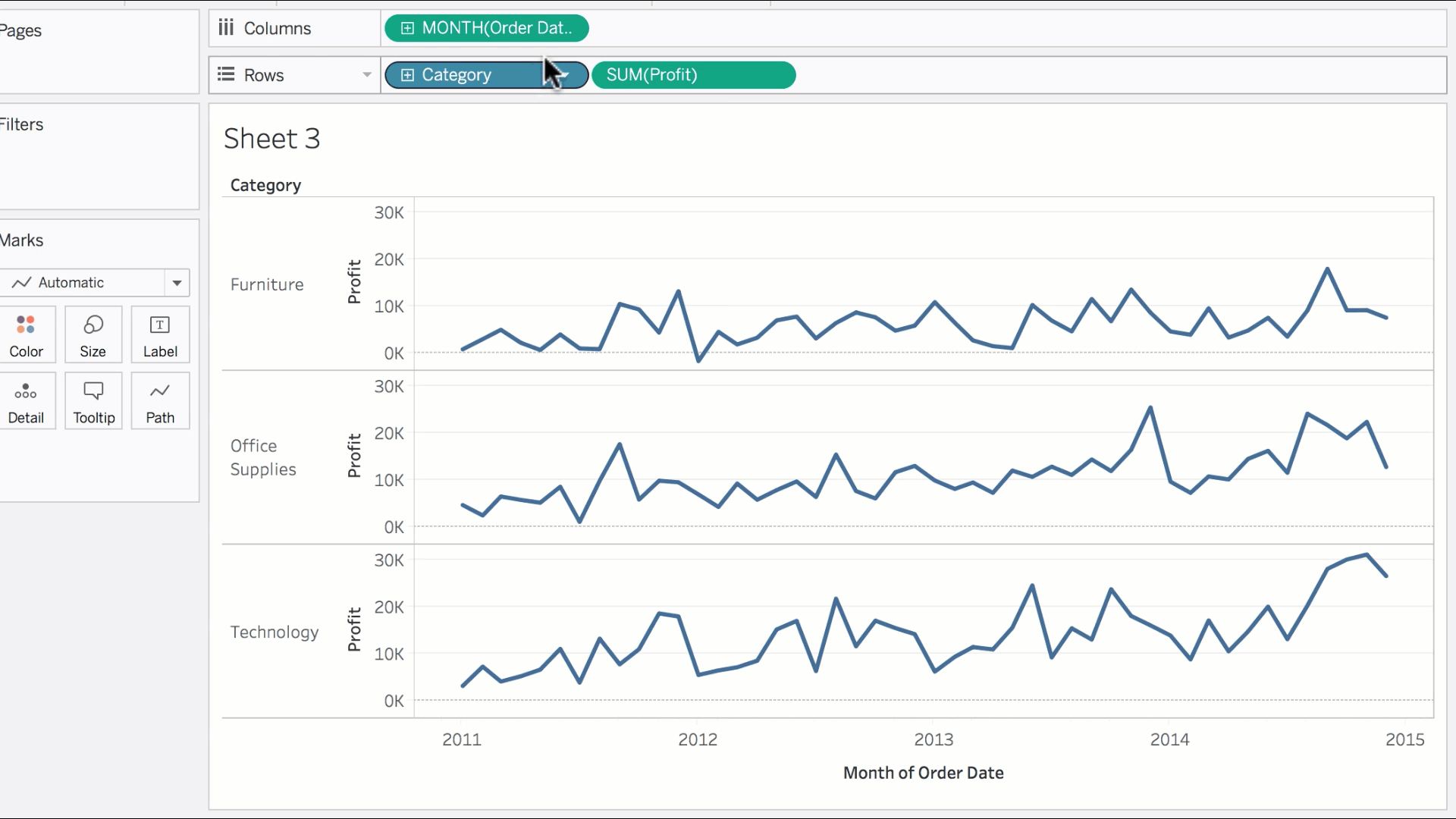Feedback Summary
5.0
1 students
5

100%
4

0%
3

0%
2

0%
1

0%
Written Reviews
There are no written reviews yet.